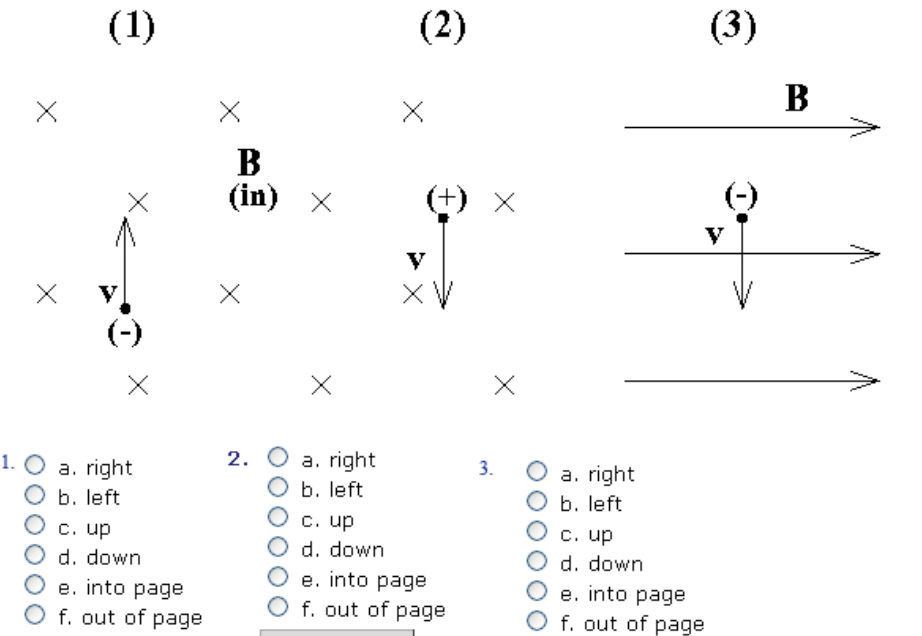In: Physics

For questions 1-3 use the figure below and give the direction of the magnetic force on the charged particle (black dot).

For questions 1-3 use the figure below and give the direction of the magnetic force on the charged particle (black dot). Note that for 1 and 2 the B-field is into the page and for 3 it is to the right. Note also the sign of the charge on the particle is given in parentheses (+) or (-).Solutions

Expert Solution

The magnetic force is given by the Lorentz force law and is

F = Q(v x B)

Where the right-hand rule gives the direction of the cross-product.

1) For a particle (1), the right-hand rule gives a cross-product direction to the right (point your index finger of your right hand along the velocity vector of the particle and curl your middle finger in the direction of the magnetic field, and your thumb gives the direction of the force.) But because the charge of the particle is negative (i.e., Q = -q), the negative sign in front of the Lorentz force law for this particle (F =-q(v x B)) will give a vector in the opposite direction (to the right), so our answer is (a)right.

2) For a particle (2), the right-hand rule gives a direction to the right. The charge on particle 2 is positive, so the Lorentz force law just gives us a force in the direction given by the righthand rule. So our answer is (a) right.

3) For a particle (3), the right-hand rule gives us a direction out of the page, but because the charge on particle 3 is negative, the Lorentz force law gives us a force in the opposite direction our answer is e) into the page.

Note that the answers I have given are the instantaneous forces on the particles and will not be correct the instant after the magnetic force has changed the direction of these particles' velocities. Because the magnetic fields in this problem are uniform, the particles will have a continuous force on them directed perpendicular to their velocity vector to be continuously deflected in that direction. This type of force is identical to centripetal acceleration, so the particles will continue in a uniform circular motion as long as the field remains uniform.

Related Solutions

Determine the magnitude and direction of the electric field at point 1 in the figure(Figure 1).
Figure 1 Part A Determine the magnitude and direction of the electric field at point 1 in the figure(Figure 1). E1→=(2500V/m,up) E1→=(7500V/m,up) E1→=(3750V/m,down) E1→=(2500V/m,down) Part B Determine the magnitude and direction of the electric field at point 2 in the figure. E2→=(2500V/m,up) E2→=(3750V/m,down) E2→=(7500V/m,down) E2→=(5000V/m,up)
Watch this video and then use the figure below to answer the question
Watch this video and then use the figure below to answer the questions. Part A Match each component of the electron transport chain with its description. Drag the terms on the left to the appropriate blanks on the right to complete the sentences.
What is the magnetic field at the center of the loop in the figure? (figure 1)
Figure 1 What is the magnetic field at the center of the loop in the figure? (Figure 1)
What are the magnitude and direction of the current in the 20 Ω resistor in (Figure 1)?
(Figure 1) What are the magnitude and direction of the current in the 20 Ω resistor in (Figure 1)? Express your answer with the appropriate units. Enter positive value if the current is clockwise and negative value if the current is counterclockwise.
What is the magnitude of the net force on the first wire in (figure 1)?
Figure 1 What is the magnitude of the net force on the first wire in (Figure 1)? What is the magnitude of the net force on the second wire in (Figure 1)? What is the magnitude of the net force on the third wire in (Figure 1)?
The loop in the figure below is being pushed into the 0.20 T magnetic field at 50 m/s.
The loop in the figure below is being pushed into the 0.20 T magnetic field at 50 m/s. The resistance of the loop is 0.10 , and its width d = 5.4 cm. What are the direction and the magnitude of the current in the loop? (in units of A)
In (Figure 1), charge q2 experiences no net electric force. What is q1?
In (Figure 1), charge q2 experiences no net electric force. What is q1?